PSABM5ZY - Practice---Acc7.5 Lesson 21 The Slope of a Fitted Line (8.EE.B.6, 8.SP.A.1, 8.SP.A.2, 8.SP.A.3)

Which of these statements is true about the data in the scatter plot?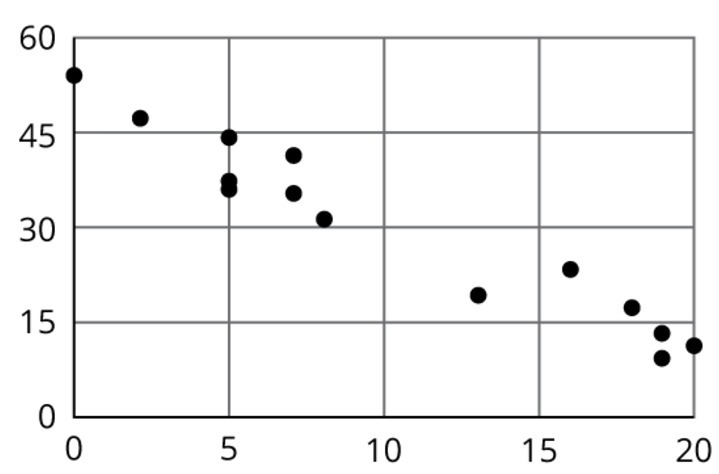Select one:

Here is a scatter plot that compares hits to at bats for players on a baseball team.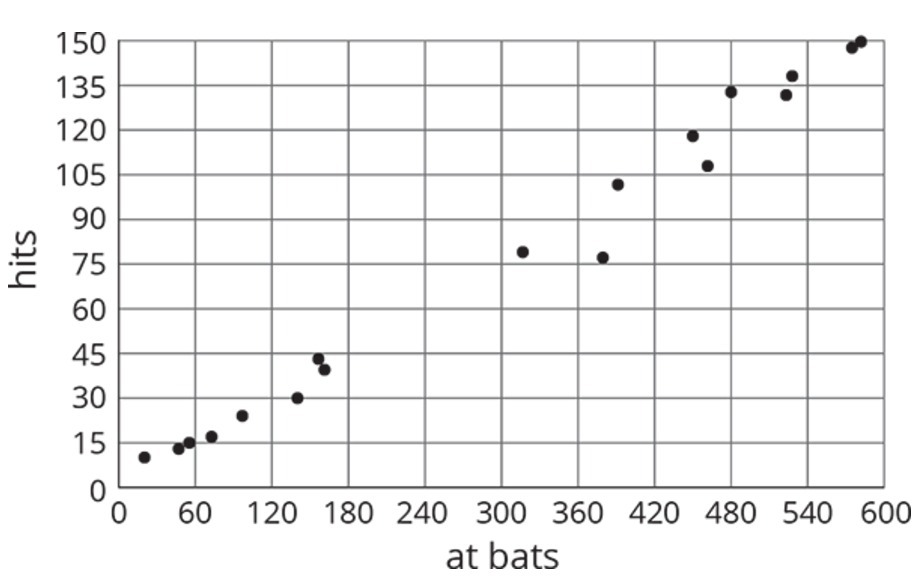Describe the relationship between the number of at bats and the number of hits using the data in the scatter plot.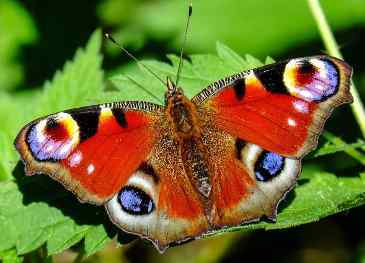The linear model for some butterfly data is given by the equation 0.2384.642. Which of the following best describes the slope of the model?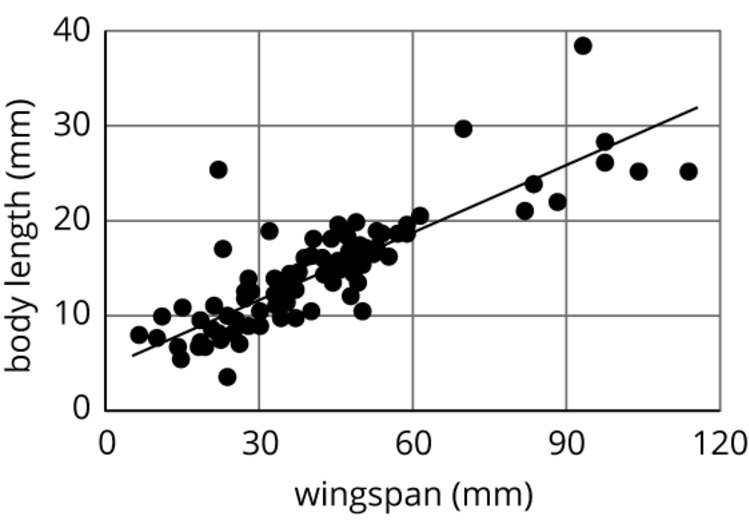Select one:

Solve:(x,y)

with no spaces

Part A)

Nonstop, one-way flight times from O’Hare Airport in Chicago and prices of a one-way ticket are shown in the scatter plot.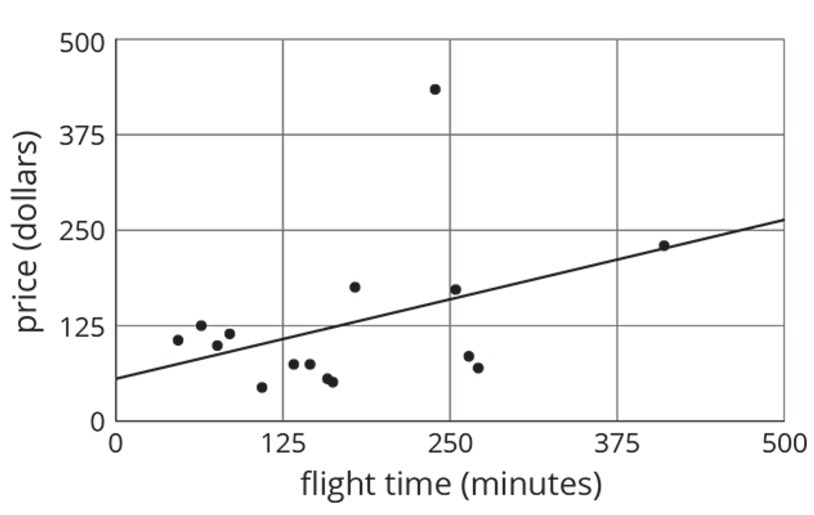Circle any data that appear to be outliers.

Indicate the data on paper, take a picture, and upload it using the image upload icon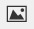If you do not have the ability to upload an image of your work type "Indicated data is on paper."

Part B)

Use the graph to estimate the difference between any outliers and their predicted values.

Part A)

Consider the following graphs of linear equations. Decide which line has a positive slope, and which has a negative slope. Then calculate each line’s exact slope.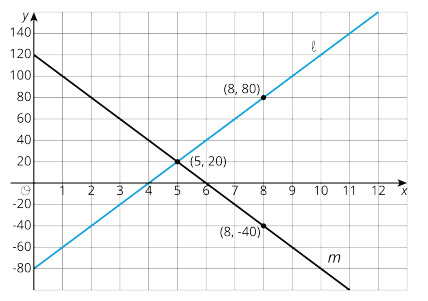Is the slope of linepositive or negative?

Select one:
Part B)

Consider the following graphs of linear equations. Decide which line has a positive slope, and which has a negative slope. Then calculate each line’s exact slope.Is the slope of line m positive or negative?

Type your answer below as a number (example: 5, 3.1, 4 1/2, or 3/2):
Part C)

Consider the following graphs of linear equations. Decide which line has a positive slope, and which has a negative slope. Then calculate each line’s exact slope.The slope for Line= _____

Type your answer below as a number (example: 5, 3.1, 4 1/2, or 3/2):
Part D)

Consider the following graphs of linear equations. Decide which line has a positive slope, and which has a negative slope. Then calculate each line’s exact slope.